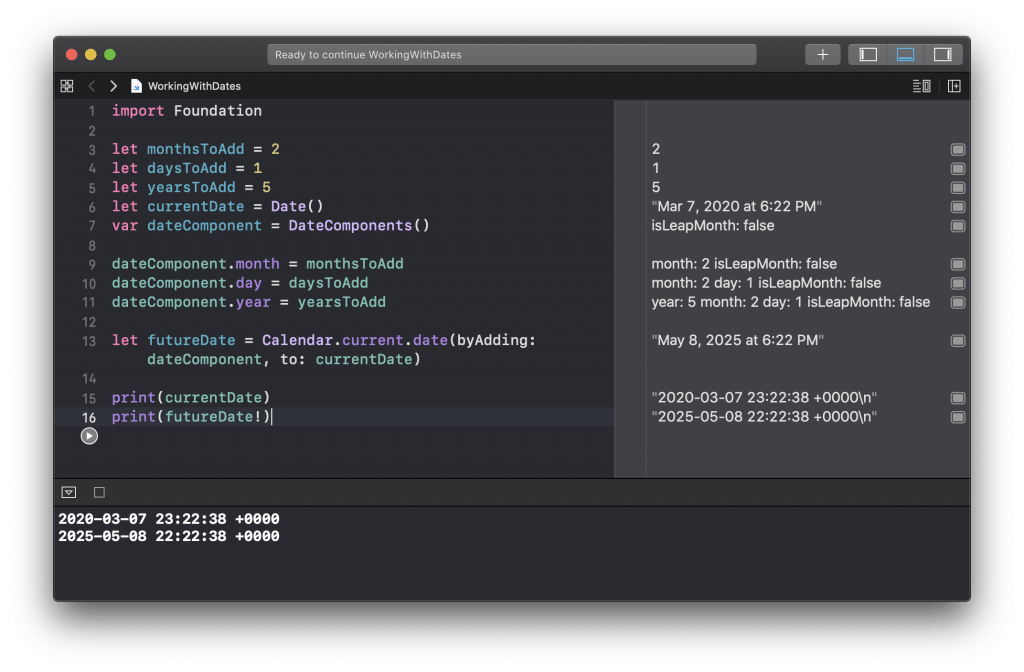# Add Days, Months, Years to Current Date in Swift

In this tutorial, you will learn how to add days, months or years to the current date in Swift.

To work with dates I will use Date(), DateComponents() and Calendar objects. I will first get the today’s current date and then will use the DateComponents() to add to a current date another day, month or a year.

## Get Current Date

To get the current date in Swift is very easy. To do that we simply need to create a new instance of a Date() object.

```let currentDate = Date()
print(currentDate)```

The output will be in the following format.

`2020-03-07 22:58:37 +0000`

## Add Days to Current Date

To add more days to a current date object, you can use the Calendar and the DateComponents() Swift structs.

```let currentDate = Date()
var dateComponent = DateComponents()
dateComponent.day = 1

let futureDate = Calendar.current.date(byAdding: dateComponent, to: currentDate)

print(currentDate)
print(futureDate!)```

The output will be in the following format.

```2020-03-07 23:03:40 +0000
2020-03-08 22:03:40 +0000```

Notice that the day has increased exactly by 1.

In a very similar way, we can add months and years to the current date.

## Add Months to Current Date

To add one or more months to the current date, you can use exactly the same approach as is above. First, get the current date. Then use DateComponents to set the number of months that needs to be added. Then, use Swift Calendar, to add the date component to the current date. Below is an example of how you can do it.

```let currentDate = Date()
var dateComponent = DateComponents()

dateComponent.month = 2

let futureDate = Calendar.current.date(byAdding: dateComponent, to: currentDate)

print(currentDate)
print(futureDate!)
```

If you run the above Swift code snippet in your Xcode Playground, the output will be of the following format.

```2020-03-07 23:10:30 +0000
2020-05-07 22:10:30 +0000```

Notice that the month has increased from 3 to 5.

## Add Years to Current Date

Adding more years to the current date is exactly the same way as adding more days or months. To add more years, you will use the DateComponents Swift struct and will set a number of years that need to be added.

```let currentDate = Date()
var dateComponent = DateComponents()

dateComponent.year = 5

let futureDate = Calendar.current.date(byAdding: dateComponent, to: currentDate)

print(currentDate)
print(futureDate!)
```

If you run this Swift code snippet in the Xcode playground, you will get the following output. Of course, the date will be different, depending on when this code snippet runs.

```2020-03-07 23:17:02 +0000
2025-03-07 23:17:02 +0000```

## Complete Code Example

Below are all three code snippets in a single Xcode Playground project.

```import Foundation

let currentDate = Date()
var dateComponent = DateComponents()